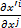• I
vibhuav
I am quite new to tensors, with my knowledge based on Daniel Fleisch’s “Student’s guide to vectors and tensors” and Neuenschwander’s “Tensor calculus for physics”. I had the following questions:

1. What are the higher rank tensors with physical meaning attached to them? I know tensors up to rank-4:
Rank-0 tensors: Scalars such as temperature etc.
Rank-1 tensors: Vectors
Rank-2 tensors: Inertia tensor, metric tensor, electromagnetic field tensor, etc.
Rank-3 tensors: Trifocal tensor
Rank-4 tensors: Riemann curvature tensor
2. (This question might not be well-formed) When are tensors necessary and when can we be satisfied with matrices (3x3, 3x3x3, 3x3x3x3)? I can understand why the tensors mentioned above – the vectors, inertia tensors, EM field tensor – should be tensors, namely to keep the tensor itself invariant upon coordinate transformation, but when would a physicist be satisfied with a matrix of numbers, without bothering about how they should transform if the coordinates are changed? I am guessing, for example, solving simultaneous equations using matrix method need not be tensors because presumably the numbers involved are without units? Would that mean that if the numbers involved were, say, pressure/unit length, we would have to make sure the matrix of numbers transforms correctly in a new coordinate system? The transformation matrix itself,, being without units, need not be tensors for that reason?

Thanks a lot.

#### Attachments

Mentor
2022 Award
I am quite new to tensors, with my knowledge based on Daniel Fleisch’s “Student’s guide to vectors and tensors” and Neuenschwander’s “Tensor calculus for physics”. I had the following questions:

1. What are the higher rank tensors with physical meaning attached to them? I know tensors up to rank-4:
Rank-0 tensors: Scalars such as temperature etc.
Rank-1 tensors: Vectors
Rank-2 tensors: Inertia tensor, metric tensor, electromagnetic field tensor, etc.
Rank-3 tensors: Trifocal tensor
Rank-4 tensors: Riemann curvature tensor
As you might have recognized, the rank of a tensor reflects the number of underlying vector spaces. So whenever you deal with more vector spaces in number than four, tensors of higher ranks might play a role. As those objects cannot really be imagined, they become more and more a mathematical tool rather than an obvious physical quantity.
2. (This question might not be well-formed) When are tensors necessary and when can we be satisfied with matrices (3x3, 3x3x3, 3x3x3x3)?
A tensor is such a number scheme, nothing else. At least if it is expressed by coordinates. The same as a linear mapping is a matrix, only that tensors are multi-linear. And that's already their actual definition: multi-linearity. But you are right to distinguish matrices and linear mappings, because the former need a basis whereas the latter could be expressed otherwise, e.g. stretching by a factor two or a reflection. It's the same with tensors. As soon as we fix a basis, we have such a multi-dimensional number scheme. If we don't have such a basis, it might become difficult to describe a tensor instead. Tensor spaces have a universal mapping property which makes them so suitable for so many situations. This means, that many other mathematical objects (e.g. a bilinear algorithm, an algebra), where linearity plays a role, can be viewed as tensor spaces together with a rule, which tensors are considered equivalent.
I can understand why the tensors mentioned above – the vectors, inertia tensors, EM field tensor – should be tensors, namely to keep the tensor itself invariant upon coordinate transformation, but when would a physicist be satisfied with a matrix of numbers, without bothering about how they should transform if the coordinates are changed? I am guessing, for example, solving simultaneous equations using matrix method need not be tensors because presumably the numbers involved are without units? Would that mean that if the numbers involved were, say, pressure/unit length, we would have to make sure the matrix of numbers transforms correctly in a new coordinate system? The transformation matrix itself, View attachment 215856, being without units, need not be tensors for that reason?
You can have units or not, this is not the point. In your examples, the numbers do have units. (Multi-) Linearity is the only characteristic which is important. And the basis vectors ##\dfrac{\partial }{\partial x_i}## in your image (at least as far as I can guess) are also "units" in a way, because they measure a physical dimension (length, temperature, time, voltage, etc.). Linearity is something which is easy to calculate with, whereas non-linear equations quickly get difficult. That's why we use linear approximations like derivatives. The costs are, that they are only locally a good approximation and we have to do the calculations either in a small neighborhood of the point of interest, or find a way to compare linear approximations at different points otherwise.

Not sure, whether this will answer your question, but here is a short summary:
https://www.physicsforums.com/insights/what-is-a-tensor/

Edit: Corrected "dimension of vector space" to "number of vector spaces". The rank says how many arguments a tensor has, i.e. the number of vector spaces it is build from. Sorry for confusion.

Last edited:
vibhuav
It will take me some time to digest it, but thanks for the reply!